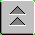[Index]

# Ring Laser Gyro

RING LASER GYRO

The Ring Laser Gyros (RLG) can be used as the stable elements (for one degree of freedom each) in an inertial guidance system. The advantage of using a RLG is that there are no moving parts. Compared to the conventional spinning gyro, this means there is no friction, which in turn means there will be no inherent drift terms. Additionally, the entire unit is compact, lightweight and virtually indestructable, meaning it can be used in aircraft.

The basic principle of operation is that a single RLG can measure any rotation about its sensitive axis. This implies that the orientation in inertial space will be known at all times. The elements that measure actual accelerations can therefore be resolved into the appropriate directions.

Here's how a RLG can measure rotation about its sensitive axis:

The input laser beam is split into two beams that travel the same path but in opposite directions: one clockwise and the other counter-clockwise.

The beams are recombined and sent to the output detector. In the absence or rotation, the path lengths will be the same and the output will be the total constructive interfernence of the two beams.

If the apparatus rotates, there will be a difference (to be shown later) in the path lengths travelled by the two beams, resulting in a net phase difference and destructive interference. The net signal will vary in amplitude depending on the phase shift, therefore the resulting amplitude is a measurement of the phase shift, and consequently, the rotation rate.

Now we will derive expressions for what was just discussed:

1. Amplitude of output signal: for two equal inputs (perfect beam splitter), the output voltage, Vout = Vin cos (Df/2), where Df = the phase difference of the beams upon recombination.

2. Phase difference due to path length difference. If the two paths are different by Dx, then that corresponds to a phase difference of Df = 2p/l(Dx).

3. Path length difference due to rotation. Assume the paths are circular, with radius = R. In the absence of rotation, the total path is 2pR for each beam. If the entire apparatus rotates and a constant rate (in the clockwise direction) given by w (radians per second) the two beams will travel different path lengths.

(A) Clockwise beam (beam 1): the beam will have to travel an additional amount (depending on time).

Path length 1 = 2pR + wRt1, where t1 is the total time for path 1.

Since the beam travels at the speed of light (regardless of rotation rate, a principle of special relativity), the total distance travelled by the beam in that time is ct1. In other words

ct1 = 2pR + wRt1.

We can solve for the time:

t1 = 2pR/(c - wR).

Which corresponds to intuition, that it will take longer than if there was no rotation.

(B) Counter-clockwise beam (beam 2): By a similar argument,

t2 = 2pR/(c + wR)

Note that the sign has changed in the denominator, and this will take less time that if there were no rotation.

4. Phase difference with rotation: since the beams take different times, there will be a net phase shift when they are recombined.

Dx = cDt = c (t1 - t2 )

= 2pcR{ 1/(c - wR) - 1/(c + wR) }

= 2pcR{2wR/(c2 - w2R2)}

= 4pcR2 w/(c2 - w2R2)

But, wR << c (otherwise, the outer part of the ring would be travelling a near the speed of light!)

Therefore we can neglect the contribution of that term to the denominator:

Dx ~ 4pR2w/c2.

Now, conmpute the phase shift:

Df ~ 2p/l (4pR2w/c2) = (8p2lR2/c2)w

And the resulting affect on Vout:

Vout = Vin cos{(4p2lR2/c2)w}

Since all the terms are constants, the output depends only on the rotation rate. The RLG therfore measures rotation rate about its sensitive axis.

5. Sensitivity calculation.